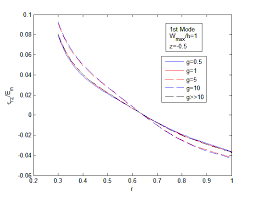## How to Calculate and Solve for Well, External and Dimensionless Radius | Nickzom CalculatorThe image above represents dimensionless radius.

To compute for the dimensionless radius, two essential parameters are needed and these parameters are external radius (re) and well radius (rw).

The formula for calculating the dimensionless radius:

rD = re / rw

Where;

Let’s solve an example;
Given that the external radius is 18 and the well radius is 16.

This implies that;

re = External Radius = 18
rw = Well Radius = 16

rD = re / rw
rD = 18 / 16
rD = 1.125

Therefore, the dimensionless radius is 1.125.

re = rD x rw

Where;

Let’s solve an example;
Given that the dimensionless radius is 9 and the well radius is 20.